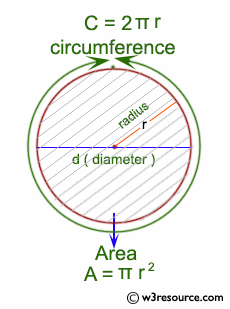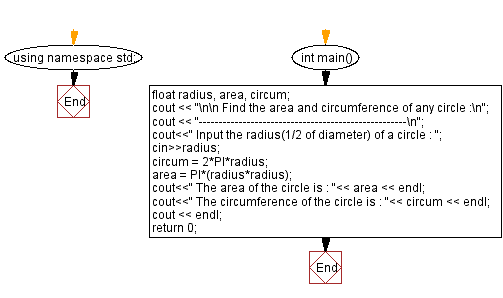﻿ C++ : Compute the area and circumference of a circle

# C++ Exercises: Accepts the radius of a circle from the user and compute the area and circumference

## C++ Basic: Exercise-43 with Solution

Write a C++ program that accepts the radius of a circle from the user and computes the area and circumference.

Visual Presentation:Sample Solution:

C++ Code :

``````#include <iostream> // Including the input-output stream header file

#define PI 3.14159 // Defining a constant PI with the value 3.14159

using namespace std; // Using the standard namespace

int main() // Start of the main function
{
float radius, area, circum; // Declaring variables to store radius, area, and circumference

cout << "\n\n Find the area and circumference of any circle :\n"; // Outputting a message indicating the purpose of the program
cout << "----------------------------------------------------\n"; // Displaying a separator line

cout << " Input the radius(1/2 of diameter) of a circle : "; // Prompting the user to input the radius of the circle

circum = 2 * PI * radius; // Calculating the circumference of the circle using the formula: 2 * PI * radius
area = PI * (radius * radius); // Calculating the area of the circle using the formula: PI * (radius^2)

cout << " The area of the circle is : " << area << endl; // Outputting the calculated area of the circle
cout << " The circumference of the circle is : " << circum << endl; // Outputting the calculated circumference of the circle

cout << endl; // Displaying an empty line for better readability
return 0; // Return statement indicating successful completion of the program
}
``````

Sample Output:

```Find the area and circumference of any circle :
----------------------------------------------------
Input the radius(1/2 of diameter) of a circle : 5
The area of the circle is : 78.5397
The circumference of the circle is : 31.4159
```

Flowchart:C++ Code Editor:

What is the difficulty level of this exercise?

﻿